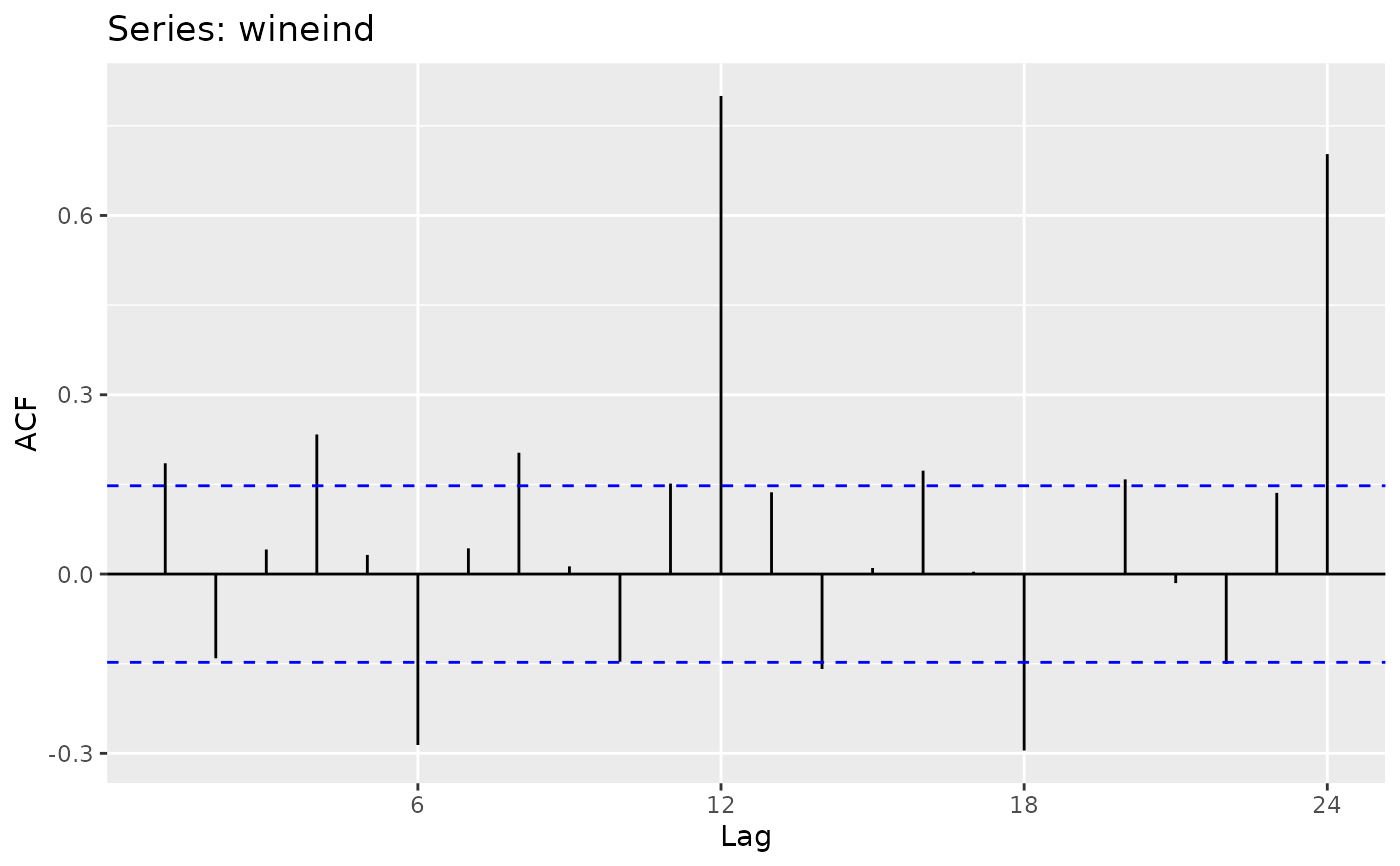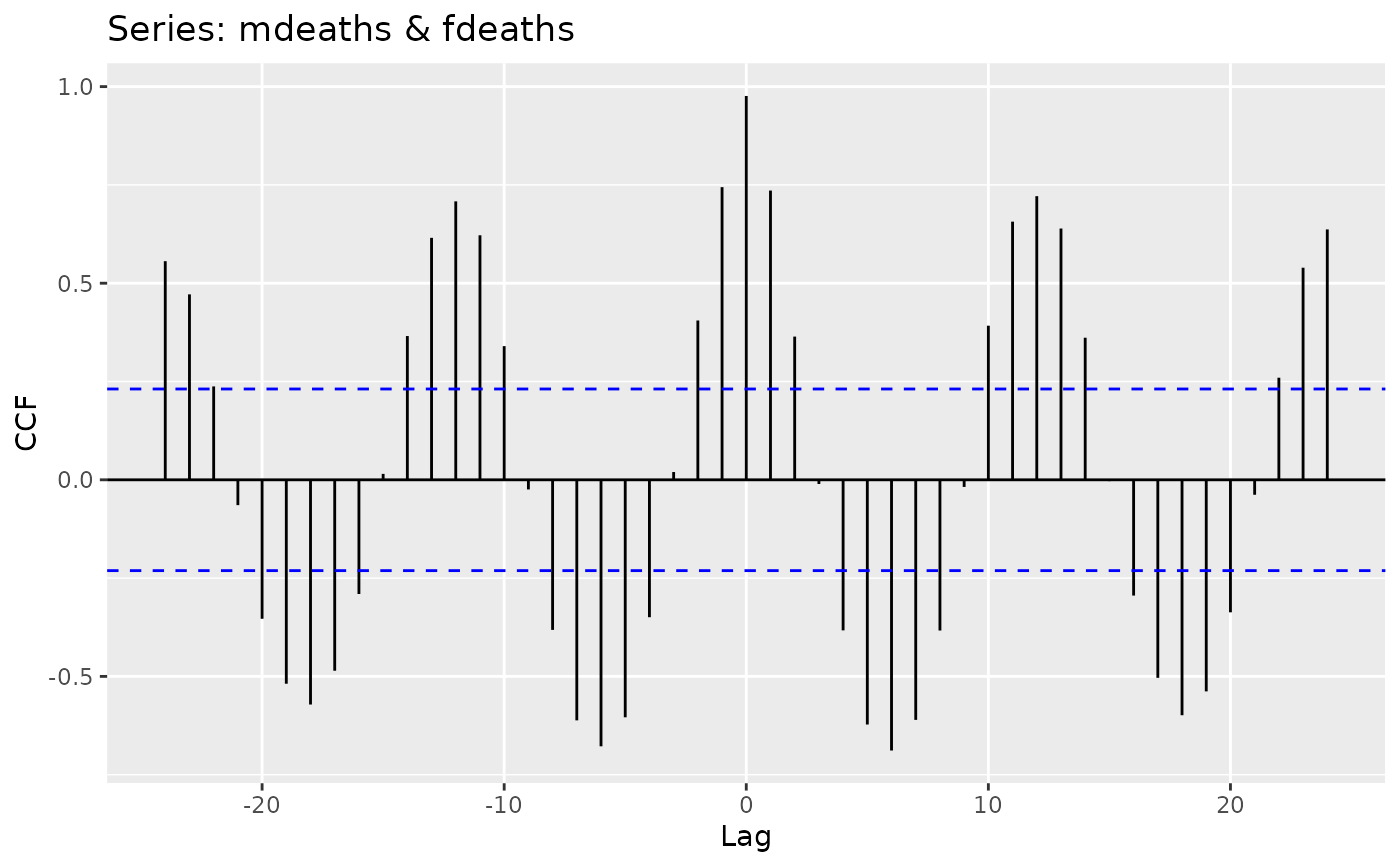Produces a ggplot object of their equivalent Acf, Pacf, Ccf, taperedacf and taperedpacf functions.

## Usage

# S3 method for acf
autoplot(object, ci = 0.95, ...)

ggAcf(
x,
lag.max = NULL,
type = c("correlation", "covariance", "partial"),
plot = TRUE,
na.action = na.contiguous,
demean = TRUE,
...
)

ggPacf(
x,
lag.max = NULL,
plot = TRUE,
na.action = na.contiguous,
demean = TRUE,
...
)

ggCcf(
x,
y,
lag.max = NULL,
type = c("correlation", "covariance"),
plot = TRUE,
na.action = na.contiguous,
...
)

# S3 method for mpacf
autoplot(object, ...)

ggtaperedacf(
x,
lag.max = NULL,
type = c("correlation", "partial"),
plot = TRUE,
calc.ci = TRUE,
level = 95,
nsim = 100,
...
)

ggtaperedpacf(x, ...)

## Arguments

object

Object of class “acf”.

ci

coverage probability for confidence interval. Plotting of the confidence interval is suppressed if ci is zero or negative.

...

Other plotting parameters to affect the plot.

x

a univariate or multivariate (not Ccf) numeric time series object or a numeric vector or matrix.

lag.max

maximum lag at which to calculate the acf.

type

character string giving the type of acf to be computed. Allowed values are "correlation" (the default), “covariance” or “partial”.

plot

logical. If TRUE (the default) the resulting ACF, PACF or CCF is plotted.

na.action

function to handle missing values. Default is na.contiguous. Useful alternatives are na.pass and na.interp.

demean

Should covariances be about the sample means?

y

a univariate numeric time series object or a numeric vector.

calc.ci

If TRUE, confidence intervals for the ACF/PACF estimates are calculated.

level

Percentage level used for the confidence intervals.

nsim

The number of bootstrap samples used in estimating the confidence intervals.

A ggplot object.

## Details

If autoplot is given an acf or mpacf object, then an appropriate ggplot object will be created.

ggtaperedpacf

plot.acf, Acf, acf, taperedacf

## Author

Mitchell O'Hara-Wild

## Examples


library(ggplot2)
ggAcf(wineind)wineind %>% Acf(plot=FALSE) %>% autoplotif (FALSE) {
wineind %>% taperedacf(plot=FALSE) %>% autoplot
ggtaperedacf(wineind)
ggtaperedpacf(wineind)}
ggCcf(mdeaths, fdeaths)